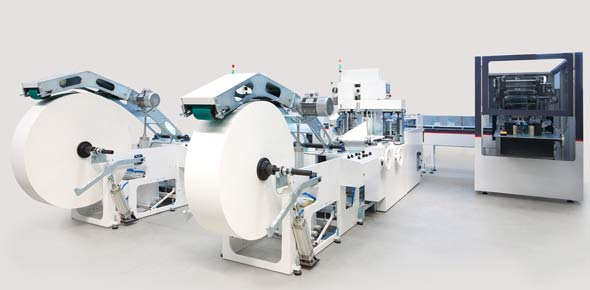# Work And Simple Machines Block 1

20 Questions | Total Attempts: 40SettingsThis quiz is designed to be used as an assessment following the use of my multimedia website.

• 1.
The transfer of energy that occurs when a force makes an object move a distance.
• A.

Force

• B.

Work

• C.

Power

• D.

Joule

• 2.
A baseball bat is an example of this class of lever:
• A.

First-class

• B.

Second-class

• C.

Third-class

• 3.
SI unit for power
• A.

Joule

• B.

Watt

• C.

Newton

• 4.
All of these are examples of inclined planes except:
• A.

Slide

• B.

Ramp

• C.

Staircase

• D.

Wheel

• 5.
The amount of work done per unit of time
• A.

Power

• B.

• C.

Work

• D.

Force

• 6.
The number of times a machine multiplies an effort force.
• A.

Energy

• B.

• C.

Input force

• D.

Output force

• 7.
Which is not an example of a wedge?
• A.

Ax

• B.

Knife

• C.

Ramp

• D.

Scissors

• 8.
SI unit of measurement for energy and work.
• A.

Watt (W)

• B.

Newton (N)

• C.

Joule (J)

• 9.
A flag pole is an example of this type of pulley:
• A.

Fixed

• B.

Movable

• C.

Block and tackle

• D.

Pulley system

• E.

• 10.
An ax is an example of a (an)
• A.

Inclined plane

• B.

Wheel and axle

• C.

Pulley

• D.

Wedge

• 11.
Which job would be best done by a wedge?
• A.

Slicing an onion

• B.

Pulling a box

• C.

Lifting a bucket

• D.

Moving a fork

• 12.
In which of the following is no work done?
• A.

• B.

Lifting a book

• C.

Pushing on a wall

• D.

Pushing an object 3 meters

• 13.
The efficiency of a machine is always less than 100 percent because
• A.

The work input is too small

• B.

The work output is too small

• C.

A machine can't have a mechanical advantage less than 1

• D.

Some work input is lost to friction and heat

• 14.
When the kinetic energy of an object increases, which of the following also increases?
• A.

Force

• B.

Velocity

• C.

Potential energy

• D.

Gravitational pull

• 15.
Which type of pulley has the greatest mechanical advantage?
• A.

Fixed

• B.

Moveable

• C.

Compound or pulley system

• 16.
A simple machine that is a rigid bar that has to move around a fixed point called a fulcrum.
• A.

Pulley

• B.

Screw

• C.

Lever

• D.

Wheel and axle

• 17.
If a tractor pulls a trailer 600 m with a force of 1,300 N, how much work would it be doing?
• A.

780,000 W

• B.

780,000 J

• C.

780,000 N

• D.

78,000 J

• 18.
A wheel barrow is which class of lever?
• A.

First class

• B.

Second class

• C.

Third class

• 19.
The force exerted on an object by a machine may be called any of the following except.
• A.

Output force

• B.

Input force

• C.

• D.

Resistance

• 20.
If you exert a force of 10.0 N to lift a box a distance of 0.75 m, how much work do you do?
• A.

7.5 J

• B.

75 J

• C.

75 N

• D.

7.5 N

Related TopicsBack to top